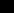CMIS 160
Discrete Mathematics
Erich Prisner
UMUC SG
Spring 2002

# A phone chain (Maximum spanning trees)

16 students sit at desks shown below. The teacher wants them to have a "phone chain" for emergencies. This is a plan of phone calls such that everybody eventually gets the news. However there are certain rules:
• First the teacher informs the student to the left of the bottom row.
• all other calls should be between adjacent students.
• the "closeness" between two adjacent students is expressed by the number of common letters. It is also called the "weight" of the edge.
• The cost of each call equals (30 - closeness), for the corresponding pair of adjacent students.
• The list should minimize the total cost, the sum of the costs of all calls.
Try the concept of closeness at some examples. Type in two names (all letters small) and compute the number of common letters. Multiple common letters are also counted several times.
the weight between and . The weight is
Now here you can generate random names (or take your own), compute the weights between the vertices, and find a maximum weight spanning tree either using single steps or all steps at once. White, yellow, orange, red, purple, and blue indicate weights of 0, 1, 2, 3, 4, and 5 or larger.0, 1, 23, 4, 5, 67, 8, 910, 11, 12, 1314, 15, 1617, 18, 19, 2021, 22, 23
Total weight = ......................... Generate names.
Maximum weight spanning tree:
With fixed names (edge weights), obviously several maximum weight spanning trees are possible. Given some (partial) tree, clicking on a yet unchosen edge may convince the computer to include it, and delete another previously chosen edge instead. But for some edges, the computer denies taking them. What does that mean for that edge? How can we find such edges?

Question: What is the expected value of the weight of an edge for the random names generated above (look at the Javascript program, and assume that the random numbers generated there are "random" enough)?

Probably difficult question: What is the expected value of the weight of a maximum spanning tree for the random names generated in this way?

The next three questions can not be answered using the applet, but try to think on them anyway:

Question: What happens if we add the requirement that each student should make at most two phone calls, one receiving and one transmitting the information (the classical phone chain)? Which graph theoretical concept do we get if we still want to minimize the total cost?

Question: What happens if we instead add the requirement that after 10 minutes everybody should be informed. Assume that each call takes exactly 1 minute.

Question: What happens if the cost of each call equals (2 - closeness), i.e. if many calls have negative cost. In that case, adjacent people with negative call cost should call each other in any case. What would the structure of the phone chain be then?

another MST page